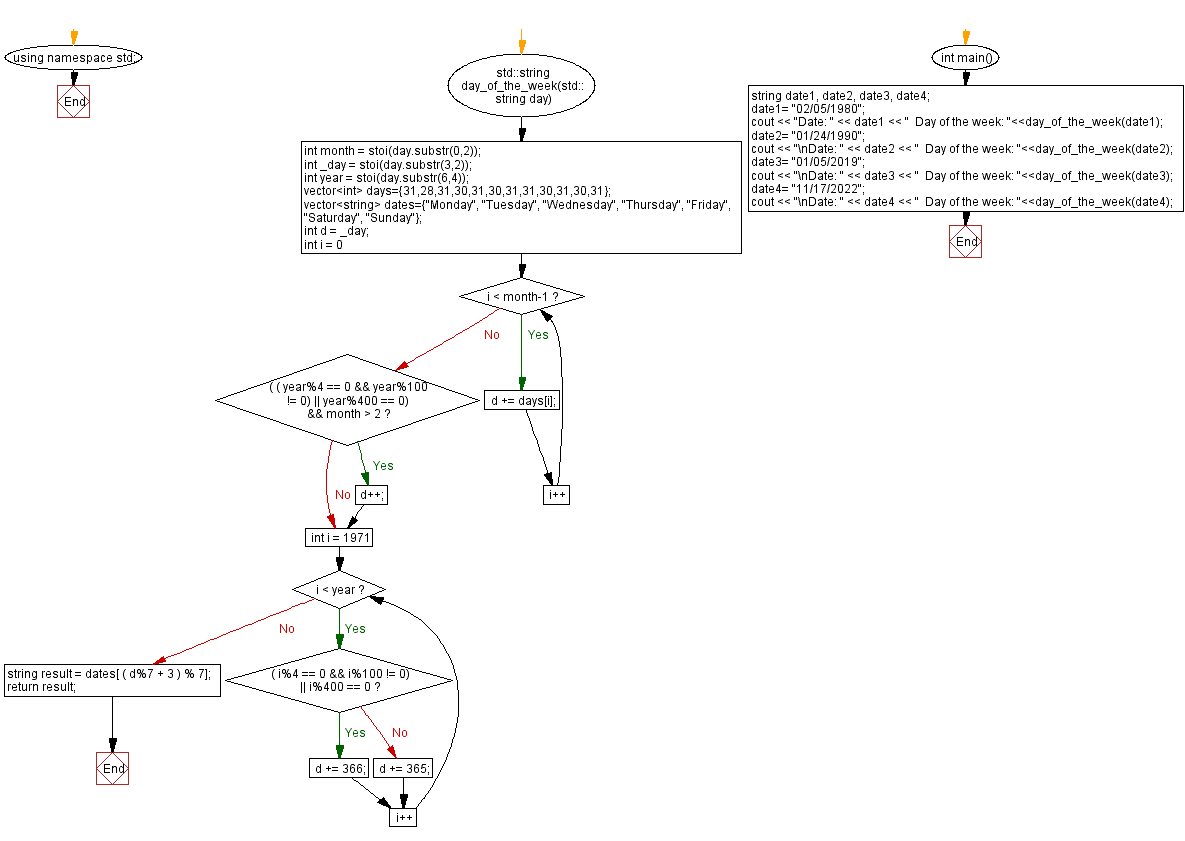﻿ C++ Exercises: Get the day of the week from a given date - w3resource

# C++ Exercises: Get the day of the week from a given date

## C++ Date: Exercise-2 with Solution

Write a C++ program to get the day of the week from a given date. Return the day as a string. The format of the day is MM/DD/YYYY.

• YYYY denotes the 4 digit year.
• MM denotes the 2 digit month.
• DD denotes the 2 digit day.

Note: Assume the week starts on Monday.

Sample Solution:

C++ Code:

``````#include <iostream>
#include <string>
#include<vector>

using namespace std;

std::string day_of_the_week(std::string day) {
int month = stoi(day.substr(0,2));
int _day = stoi(day.substr(3,2));
int year = stoi(day.substr(6,4));

vector<int> days={31,28,31,30,31,30,31,31,30,31,30,31};
vector<string> dates={"Monday", "Tuesday", "Wednesday", "Thursday", "Friday", "Saturday", "Sunday"};
int d = _day;
for(int i = 0; i < month-1; i++) d += days[i];
if ( ( ( year%4 == 0 && year%100 != 0) || year%400 == 0) && month > 2) d++;
for(int i = 1971; i < year; i++) {
if ( ( i%4 == 0 && i%100 != 0) || i%400 == 0) d += 366;
else d += 365;
}

string result = dates[ ( d%7 + 3 ) % 7];
return result;
}
int main(){
string date1, date2, date3, date4;
date1= "02/05/1980";
cout << "Date: " << date1 << "  Day of the week: "<<day_of_the_week(date1);
date2= "01/24/1990";
cout << "\nDate: " << date2 << "  Day of the week: "<<day_of_the_week(date2);
date3= "01/05/2019";
cout << "\nDate: " << date3 << "  Day of the week: "<<day_of_the_week(date3);
date4= "11/17/2022";
cout << "\nDate: " << date4 << "  Day of the week: "<<day_of_the_week(date4);
}

``````

Sample Output:

```Date: 02/05/1980  Day of the week: Tuesday
Date: 01/24/1990  Day of the week: Wednesday
Date: 01/05/2019  Day of the week: Saturday
Date: 11/17/2022  Day of the week: Thursday
```

N.B.: The result may vary for your current system date and time.

Flowchart:C++ Code Editor: